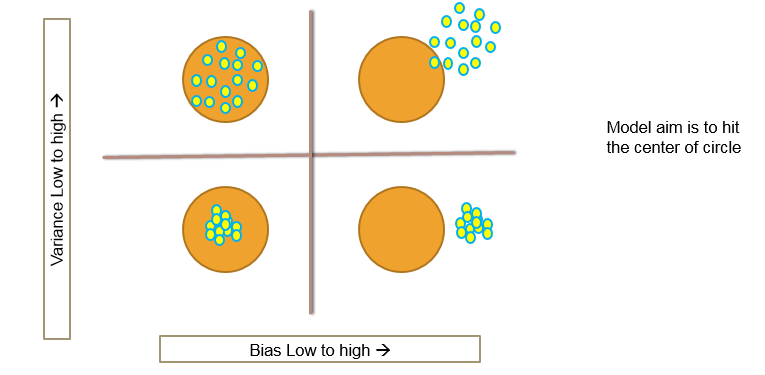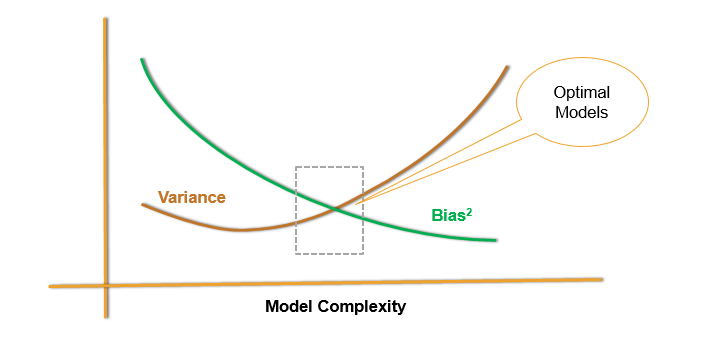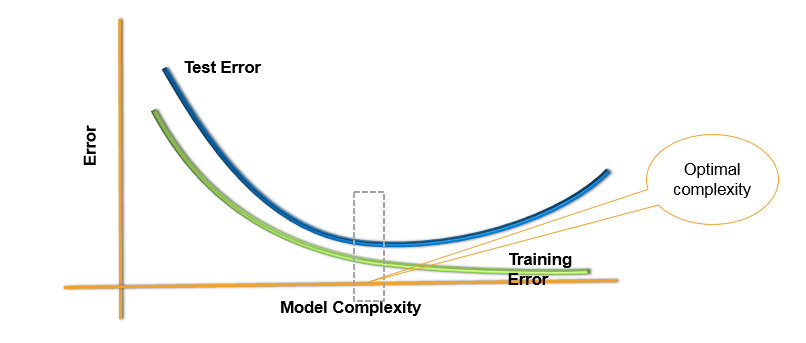• No products in the cart.

### Model Bias and Variance

In previous section, we studied about Type of Datasets, Type of Errors and Problem of Overfitting

• Over fitting
• Low Bias with High Variance
• Low training error – ‘Low Bias’
• High testing error
• Unstable model – ‘High Variance’
• The coefficients of the model change with small changes in the data
• Under fitting
• High Bias with low Variance
• High training error – ‘high Bias’
• testing error almost equal to training error
• Stable model – ‘Low Variance’
• The coefficients of the model doesn’t change with small changes in the data### The Bias-Variance Decomposition

$Y = f(X)+\epsilon$ $Var(\epsilon) = \sigma^2$ $Squared Error = E[(Y -\hat{f}(x_0))^2 | X = x_0 ]$ $= \sigma^2 + [E\hat{f}(x_0)-f(x_0)]^2 + E[\hat{f}(x_0)-E\hat{f}(x_0)]^2$ $= \sigma^2 + (Bias)^2(\hat{f}(x_0))+Var(\hat{f}(x_0 ))$

Overall Model Squared Error = Irreducible Error + $Bias^2$ + Variance

### Bias-Variance Decomposition

• Overall Model Squared Error = Irreducible Error + $Bias^2$ + Variance
• Overall error is made by bias and variance together
• High bias low variance, Low bias and high variance, both are bad for the overall accuracy of the model
• A good model need to have low bias and low variance or at least an optimal where both of them are jointly low
• How to choose such optimal model. How to choose that optimal model complexity

### Choosing optimal model-Bias Variance Tradeoff### Test and Training ErrorThe next post is about Cross validation.

21st June 2017

### 0 responses on "203.4.6 Model-Bias Variance Tradeoff"

Statinfer Software Solutions LLP

Software Technology Parks of India,
NH16, Krishna Nagar, Benz Circle,